Wang, J., Zeng, B., Chen, J., Zhan, P., and Zhang, Y. (2020). "Combination of xylanase and cellulase hydrolysis for enhanced saccharification of poplar chips: Process optimization," BioRes. 15(1), 840-853.

#### Abstract

Removing xylan from the outer surface of cellulose could promote the accessibility of cellulase to cellulose. Under this premise, in order to develop an efficient pretreatment method to obtain fermentable reducing sugar from poplar fiber, a response surface methodology was created via the combination of the Plackett-Burman design and the Box-Behnken design and was utilized by the Design-Expert software. The optimum conditions were as follows: a liquid-solid ratio of 4.95 to 1 (mL/g), a pre-hydrolysis xylanase temperature of 70.3 °C, and a total hydrolysis time of cellulase of 52.9 h. Under these conditions, the maximum amount of reducing sugars converted from poplar fiber was 159.6 mg/g. The average amount from the validation results was 160.1 mg/g, which was in agreement with the predicted value. Meanwhile, the poplar fiber hydrolyzed via a xylanase-aided two-stage pretreatment was compared with the poplar fiber hydrolyzed via only a cellulase pretreatment, using scanning electron microscopy. The xylanase-aided treatment revealed a surface with a more damaged structure, indicating that the utilization of xylanase could further improve the effects of hydrolysis on poplar fibers.

Combination of Xylanase and Cellulase Hydrolysis for Enhanced Saccharification of Poplar Chips: Process Optimization

Junying Wang,a,b Baiquan Zeng,a,b,* Jienan Chen,b,c Peng Zhan,b,c and Yangyang Zhang d

Removing xylan from the outer surface of cellulose could promote the accessibility of cellulase to cellulose. Under this premise, in order to develop an efficient pretreatment method to obtain fermentable reducing sugar from poplar fiber, a response surface methodology was created via the combination of the Plackett-Burman design and the Box-Behnken design and was utilized by the Design-Expert software. The optimum conditions were as follows: a liquid-solid ratio of 4.95 to 1 (mL/g), a pre-hydrolysis xylanase temperature of 70.3 °C, and a total hydrolysis time of cellulase of 52.9 h. Under these conditions, the maximum amount of reducing sugars converted from poplar fiber was 159.6 mg/g. The average amount from the validation results was 160.1 mg/g, which was in agreement with the predicted value. Meanwhile, the poplar fiber hydrolyzed via a xylanase-aided two-stage pretreatment was compared with the poplar fiber hydrolyzed via only a cellulase pretreatment, using scanning electron microscopy. The xylanase-aided treatment revealed a surface with a more damaged structure, indicating that the utilization of xylanase could further improve the effects of hydrolysis on poplar fibers.

Keywords: Poplar fiber; Xylanase-aided two-stage pretreatment; Reducing sugar; Plackett-Burman design; Box-Behnken design

Contact information: a: College of Life Science and Technology, Central South University of Forestry & Technology, Changsha, 410004 China; b: Ministry of Forestry Bioethanol Research Center, Changsha, 410004 China; c: Hunan Engineering Research Center for Woody Biomass Conversion, Changsha 410004 China; d: College of Food Science, XinYang College of Agriculture And Forestry, Xinyang, 464000 China; * Corresponding author: baiquanzhn@163.com

INTRODUCTION

A series of problems, including the continuous large consumption of fossil fuel, global warming, as well as environmental pollution, have been forcing the global scientific community to increase research in low-cost, renewable, and pollution-free energy (Rubin et al. 2008). It has been proposed that biomass can be considered one of the most promising feedstocks for green energy production (Capolupo and Faraco 2016). Lignocellulose is one of the most widely distributed and most abundant energy sources (Kim et al. 2009; Bhutto et al. 2017); it also has a low cost and natural regeneration (Coulier et al. 2013; Abdul et al. 2017). The lignocellulose can be decomposed into fermentable reducing sugar, which then could be used for the production of bioethanol. Poplar has been rated as have great potential for energy production due to its fast growth, low lignin content, and low fertilizer demand (Rekha et al. 2013). In addition, poplar is the most widely cultivated tree species with the highest wood yield in the middle latitude plain in China (Su et al. 2004). Poplar has a high cellulose content (42% to 49%), a low ash and extracts content, as well as moderate hemicellulose (16% to 23%) and lignin (21% to 29%) content (Sannigrahi et al. 2010). These characteristics make poplar an ideal raw material for various biomass material applications. As a result, the development and utilization of poplar residues are of great importance to the economy and ecology.

Pretreatment is an important step for the utilization of lignocellulose, in which the material can be converted into fermentable sugars (Bondesson and Galbe 2016). Generally, there are physical methods, chemical methods, and combinations to pretreat lignocellulose. The purpose of a pretreatment is to change the properties of the lignocellulosic material for the preparation of the enzymatic degradation (Taherzadeh and Karimi 2008). Compared to an alkaline pretreatment, acid pretreatment has a higher yield of sugar in its monomeric form (Yang and Wyman 2008). Steam explosion is an effective physical pretreatment, which improves the accessibility between the cellulose and enzymes (Cantarella et al. 2004). Cellulase can hydrolyze cellulose via the synergistic effect of the constituent enzymes found in cellulase. However, the presence of hemicellulose blocks the hydrolysis of the cellulase enzymes to the cellulose. As the main component of hemicellulose, xylan forms a sheath on the surface of cellulose (Pauly et al. 2000). Thus, the removal of the “sheath” can improve accessibility of cellulose to the cellulase enzyme. In the present study, xylanase-aided two-stage pretreatment was utilized. It was a combined treatment that consists of an initial xylanase hydrolysis followed by cellulases hydrolysis. Utilization of xylanase can assist in the hydrolysis of cellulose, which can effectively improve the conversion rate of reducing sugars. Hu et al. (2011) had observed the phenomenon of xylanase boosting the conversion rate during the pretreatment of cellulose. Long et al. (2018) pre-hydrolyzed lignocellulose with xylanase from T. thermaru DSM 5069, and the results demonstrated it benefited the hydrolysis process.

The combination of the Plackett-Burman design and the Box-Behnken design could make the optimization experiments more efficient (Li et al. 2016), and it could save a lot of manpower, financial resources, material resources and time. In the present research, three significant factors were screened via Plackett-Burman design, and then were optimized by Box-Behnken design. After the process, the optimum levels of the three factors and the maximum amount of reducing sugars converted from poplar fiber could be obtained.

EXPERIMENTAL

Materials

The poplar that was crushed into chips (1 mm to 2 mm in diameter and 2 mm to 15 mm in length) was provided by a poplar plantation (Hanshou County, Changde, Hunan Province, China). Commercial cellulase (222.98 FPU, 4.5~6.0 in the optimized pH) and commercial xylanase (997 IU/mL, 4.0~6.0 in the optimized pH) was purchased from Ningxia Xiasheng Industrial Group Co., Ltd. (Yinchuan, Ningxia Province, China). Xylanase activity was determined by standard QB/T 4483-2013 (2013), and cellulase activity was determined by standard QB 2583-2003 (2013).

Methods

Pretreatment of poplar fiber

After dipping the poplar chip in a phosphoric acid solution for 60 min and undergoing steam-explosion with conditions of 2.5 MPa for 180 s, it was hydrolyzed with xylanase and cellulase at a pH of 4.8 (± 0.02), using different times (1 h to 2.5 h for the xylanase pre-hydrolysis time and 6 h to 48 h for the cellulase hydrolysis time) and temperatures (60 °C to 80 °C for the xylanase pre-hydrolysis temperature and 40 °C to 60 °C for the cellulase hydrolysis temperature).

Detection of reducing sugar

The reducing sugar content was determined via the 3,5-dinitrosalicylic acid (DNS) method (Miller 1959). The glucose standard curve was drawn with the glucose concentration (x) as the abscissa and the optical density OD540 (y) as the ordinate. The regression equation of the glucose standard curve was y = 1.6069x – 0.2415 and the coefficient of determination (R2) was 0.991. The total amount of converted reducing sugars was calculated according to Chen et al. (2017), as shown in Eq. 1,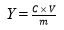(1)

where Y is the amount of converted reducing sugar (mg/g), C is the reducing sugar content (mg/mL), V is the volume of the sample (mL), and m is the quantity (g) of poplar.

Screening Experimental Parameters

The studied parameters included the effects of the liquid to solid ratio of the phosphoric acid pretreatment, the total amount of xylanase, the total xylanase pre-hydrolysis time, the xylanase pre-hydrolysis temperature, the total amount of cellulase, the total cellulase hydrolysis time, and the cellulase hydrolysis temperature on the total amount of converted reducing sugars.

Plackett-Burman design

The Plackett-Burman design (PBD) was proposed by Plackett and Burman in 1946. It is based on the incomplete equilibrium plate principle, stated by Placket et al. (1946), in which several important factors can be selected from multiple factors to facilitate further analysis. The PBD usually relates multiple parameters but typically utilizes only a few runs (Asfaram et al. 2016b). In the design, the interactions among variables are generally neglected, so the first-order model can be described by Eq. 2,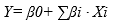(2)

where Y is the predict target response (total amount of converted reducing sugars), β0 is the intercept of the model, βi is the regression coefficient, and Xi is an independent parameter.

By using Design-expert software (Version 8.0.6, Stat-Ease, Inc., Minneapolis, MN), the liquid to solid ratio of phosphoric acid to poplar, the amount of xylanase added, the total xylanase pre-hydrolysis time, the xylanase pre-hydrolysis temperature, the amount of cellulase added, the cellulase hydrolysis temperature, and the total cellulase hydrolysis time were analyzed. The parameters and the results are shown in Table 1.

Box-Behnken design

After the PBD, the Box-Behnken design (BBD) was used to further optimize the total amount of converted reducing sugars. By using the BBD, the potential interactions between the factors could be determined (Ferreira et al. 2007). The three independent variables were the liquid-solid ratio (X1), the xylanase pre-hydrolysis temperature (X2), and the total cellulase hydrolysis time (X3). Their ranges were 4 to 1 mL/g to 6 to 1 mL/g, 65 °C to 75 °C, and 36 h to 60 h, respectively. There were 17 random experiments conducted, according to a tree-factor-three-level test. The equation of the RSM model is shown in Eq. 3,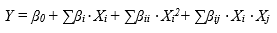(3)

where Y is the predicted response, β0 is regression coefficients of the intercept, βi is the linear coefficient, βii and βij are the quadratic coefficient and the ijth interaction coefficient, respectively, and Xi and Xj represent the coded independent variables. The design of the experiment and the regression analysis of the data were both accomplished by exploiting the Design-Expert software.

Table 1. Plackett-Burman Design and Experimental Resultsa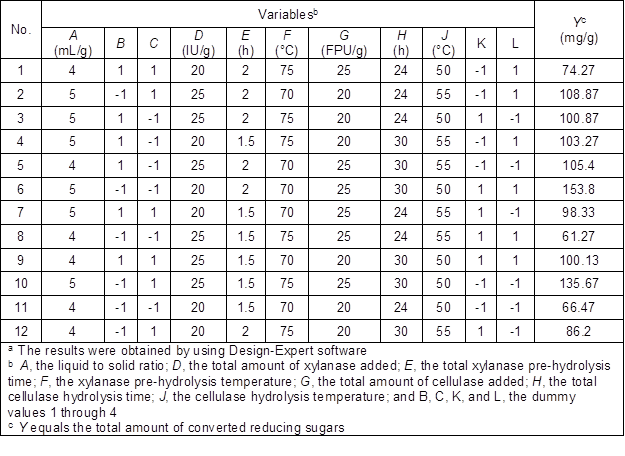RESULTS AND DISCUSSION

Screening Experimental Parameters

Effect of the liquid to solid ratio on the total amount of converted reducing sugars

As shown in Fig. 1a, as the liquid to solid ratio was gradually increased, the total amount of converted reducing sugars also increased, and it reached a maximum of 146.7 mg/g with a 5 to 1 ratio (mL/g). However, the growth rate of the amount of converted reducing sugars gradually decreased later, which may be due to an increase in moisture content in the poplar fiber, which hindered the infiltration of steam during the high-pressure steam explosion. This resulted in insufficient destruction of the internal structure of the poplar fiber, which subsequently led to a low-efficiency rate of the enzymatic hydrolysis. Considering the cost of phosphoric acid and the total amount of converted reducing sugars, the liquid-solid ratio of 4 to 1 (mL/g) was selected.

Effect of the total amount of xylanase on the total amount of converted reducing sugars

The total amount of converted reducing sugars was proportional to the amount of xylanase, and a maximum amount of 151.0 mg/g was reached with an addition of 30 IU/g of xylanase, as shown in Fig. 1b. When the added amount ranged from 10 IU/g to 20 IU/g, the total amount of converted reducing sugars increased. When the amount of xylanase added was 20 IU/g, the gradual increase in total amount of converted reducing sugars slowed down; therefore, an addition of 25 IU/g of xylanase was chosen for further experiments.

Effect of the total xylanase pre-hydrolysis time on the total amount of converted reducing sugars

The total amount of converted reducing sugars increased with an increase in the total xylanase pre-hydrolysis time, and the maximum amount of converted reducing sugars was 146.4 mg with a total xylanase pre-hydrolysis time of 2.5 h (as shown in Fig. 1c), but it was not a substantial increase when compared with the results of a 2.0 h pre-hydrolysis time (144.5 mg/g). As the xylanase had sufficiently pre-hydrolyzed within a certain time, too much time costing didn’t mean getting better effect. Therefore, a total xylanase pre-hydrolysis time of 2.0 h was chosen.

Effect of the xylanase pre-hydrolysis temperature on the total amount of converted reducing sugars

With an increase in the xylanase pre-hydrolysis temperature, the total amount of converted reducing sugars increased at first and then decreased. It reached a maximum value of 146.5 mg/g at 70 °C, as shown in Fig. 1d. To the best knowledge of the authors, this was the optimum temperature for maximum xylanase activity. Therefore, a xylanase pre-hydrolysis temperature of 70 °C was chosen for further experiments.

Effect of the total amount of cellulase on the total amount of converted reducing sugars

As shown in Fig. 1e, the total amount of converted reducing sugars increased with an increase in the total amount of cellulase, with a maximum amount of converted reducing sugars of 153.0 mg/g when the total amount of cellulase was 30 FPU/g. However, the total amount of converted reducing sugars did not increase much past an addition of 25 FPU/g of cellulase. Therefore, the addition of 25 FPU/g of cellulase was suitable amount for further experiments.

Effect of the total cellulase hydrolysis time on the total amount of converted reducing sugars

The total amount of converted reducing sugars increased with an increase in the total cellulase hydrolysis time, and reached a maximum value of 147.4 mg/g at 48 h. However, the total amount of converted reducing sugars did not change much after 36 h, which may be due to the destruction of the sugars via by-products formed in a later stage (Fig. 1f). Considering time costs, a total cellulase hydrolysis time of 36 h was more appropriate.

Effect of the cellulase hydrolysis temperature on the total amount of converted reducing sugars

As shown in Fig. 1g, when the cellulase hydrolysis temperature was increased, the total amount of converted reducing sugars increased at first and then decreased. The total amount of converted reducing sugars reached a maximum of 149.7 mg/g at 50 °C, which had the greatest cellulase activity rate. Consequently, a cellulase hydrolysis temperature of 50 °C was selected as the optimal condition.

Screening of Significant Factors via Plackett–Burman Design

In the optimization experiment, there were multiple factors that made this operation difficult; thus, the Plackett-Burman design was used to simplify the experimental process and screen several factors for further optimization (Chen et al. 2016). The effects of the liquid to solid ratio of phosphoric acid to poplar, the amount of xylanase added, the total xylanase pre-hydrolysis time, the xylanase pre-hydrolysis temperature, the amount of cellulase added, the cellulase hydrolysis temperature, and the total cellulase hydrolysis time were studied via the PBD. The design of the PBD and the results are shown in Table 1. As shown in Fig. 2, the liquid to solid ratio, the amount of xylanase added, the xylanase pre-hydrolysis time, the amount of cellulase added, and the total cellulase hydrolysis time were positive effects, while the cellulase hydrolysis temperature and the xylanase pre-hydrolysis temperature were negative effects. The analysis of variance (ANOVA) was shown in Table 2, and the model had an F-value of 44.79 and a R2 of 0.9874, which implied the model was significant. A p-value of 0.0012 indicated there was only a 0.12% chance that the model’s lack-of-fit was significant. Generally, a variable with a P value less than 0.05 is considered a significant parameter (Asfaram et al. 2016a). As a result, these factors were selected for further optimization: a liquid to solid ratio (A), a total cellulase hydrolysis time (H), and a xylanase pre-hydrolysis temperature (F). The final linear regression equations are shown as Eq. 4,

Y = 99.55 + 17.26+ 2.49+ 5.36– 5.95+ 5.24+ 14.53– 5.66J (4)

where A is the liquid to solid ratio, D is the total amount of xylanase added, E is the total xylanase pre-hydrolysis time, F is the xylanase pre-hydrolysis temperature, G is the total amount of cellulase added, H is the total cellulase hydrolysis time, and J is the cellulase hydrolysis temperature.

Optimization of the Significant Factors via the Response Surface Methodology

The response surface methodology (RSM), a collection of mathematical techniques, is used for solving multivariable problems (Li et al. 2016). Xu et al. (2015) proposed that a regression equation can be applied to fit the functional relation between the factors and responses with a reasonable design and ANOVA. For the present study, the final equation for the model in terms of coded factors is shown as Eq. 5,

Y=114.03+14.98A+5.82B+24.08C+1.63AB-4.02AC-1.70BC+0.49A2-20.35B2+14.70C2 (5)

where A is the liquid to solid ratio, B is the xylanase pre-hydrolysis temperature, and C is the total cellulase hydrolysis time.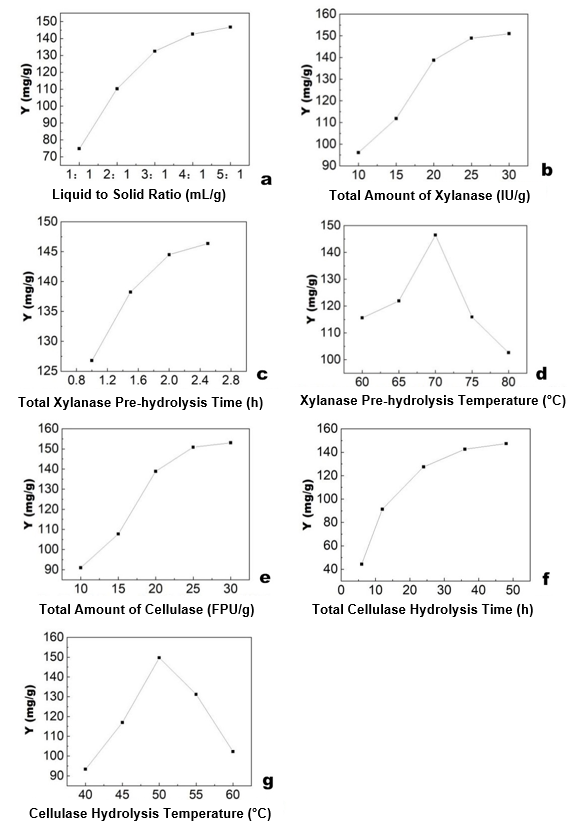Fig. 1. Different variables and the total amount of converted reducing sugars (Y)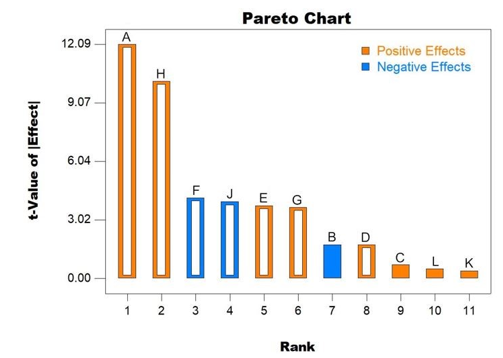Fig. 2. Pareto chart of the independent variables

Table 2. Analysis of Variance of the Plackett-Burman Designa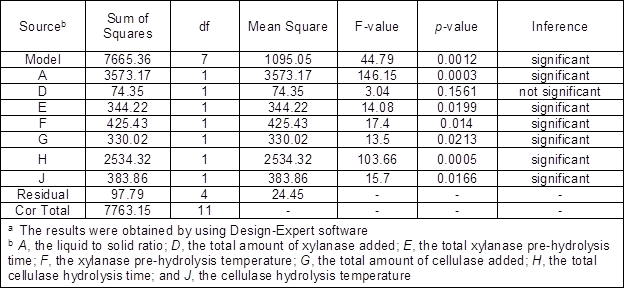Based on the results of the Plackett-Burman design, the liquid to solid ratio, the total cellulase hydrolysis time, and the xylanase pre-hydrolysis temperature were screened for further optimization using the Box-Behnken design. The results of the independent variable combination for the three parameters and the ANOVA results are shown in Tables 3 and 4, respectively. In Table 3, the results of the total amount of converted reducing sugars (Y) under different combinations were presented. As stated by Chen et al. (2015), a smaller model P-value (P-value is less than 0.05) implies that the model is significant, and a larger F-value and smaller p-value means that each of the items is significant, as stated by Asfaram et al. (2016c).

Table 3. The Box-Behnken Design and Experimental Resultsa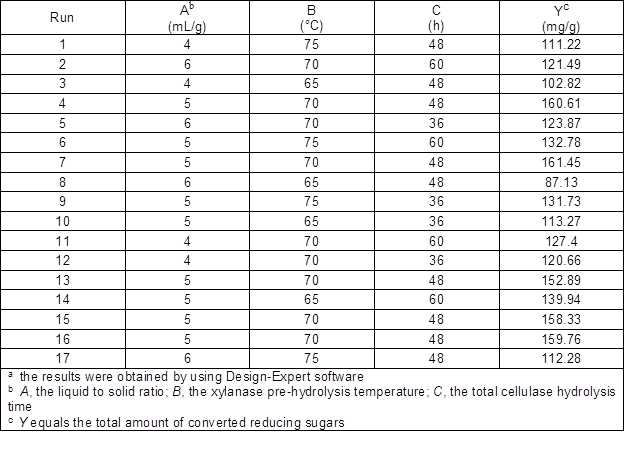Table 4. Analysis of Variance (ANOVA) of Response Surfacea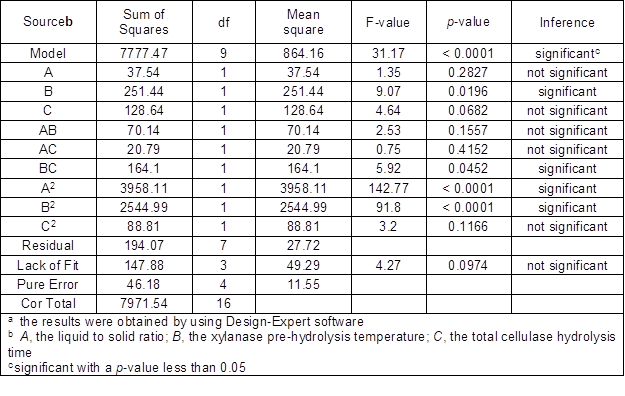As shown in Table 4, the p-values of A2 and B2 were less than 0.0001, which illustrates that their effects on the yield were significant. Meanwhile, the p-values of B and BC were less than or equal to 0.05, which implied that they were also significant. A F-value and a R2 were 216.37 and 0.9757 respectively, and a p-value was less than 0.0500, indicating that the model terms were significant as well. In addition, a lack of fit F-value of 4.27 showed that the lack of fit of the model was not significant. Therefore, this model can be used to optimize the hydrolysis of poplar fiber.

The interactions between the liquid to solid ratio (A), the xylanase pre-hydrolysis temperature (B), and the total cellulase hydrolysis time (C) are shown in Figs. 3, 4, and 5, while keeping the other variables at level 0. In Fig. 3, the response surface diagram of AC was not saddle-shaped, and the contour map of AB was close to round, indicating that the interaction between them was weak. In Figs. 4 and 5, the response surface diagram of AC and BC was saddle-shaped, meanwhile, the contour map of AC and BC were both elliptical, which indicated that the interaction between them was stronger.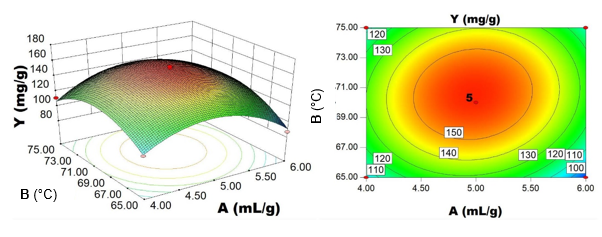Fig. 3. (left) The response surface diagram and (right) the contour map of the total amount of converted reducing sugars (Y) of the liquid to solid ratio (A) and the xylanase pre-hydrolysis temperature (B)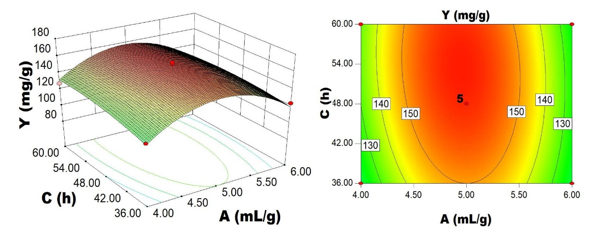Fig. 4. (left) The response surface diagram and (right) the contour map of the total amount of converted reducing sugars (Y) of the liquid to solid ratio (A) and the total cellulase hydrolysis time (C)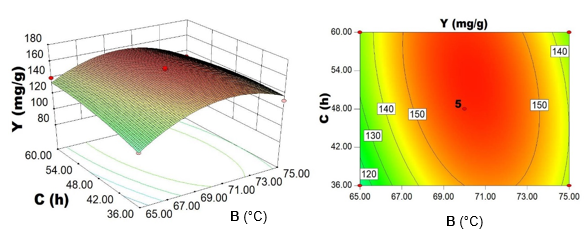Fig. 5. (left) The response surface diagram and (right) the contour map of the total amount of converted reducing sugars (Y) of the xylanase pre-hydrolysis temperature (B) and the total cellulase hydrolysis time (C)

According to Design-Expert software, the total amount of converted reducing sugars could reach a maximum value of 159.6 mg/g when the liquid to solid ratio was 4.95 to 1 mL/g, the xylanase pre-hydrolysis temperature was 70.3 °C, and the total hydrolysis time of cellulase was 52.9 h. In the actual experiment, the above conditions were adjusted to a liquid to solid ratio of 5 to 1 mL/g, a xylanase pre-hydrolysis temperature of 70.3 °C and a total cellulase hydrolysis time of 53 h. Under these conditions, the verification tests were carried out in triplicate. The average total amount of converted reducing sugars was 160.149 mg/g, which was very close to the predicted value, which illustrated that the results from the optimization of the model was accurate and reliable. It demonstrated that the response surface method was feasible to optimize a xylanase-aided two-stage pretreatment to turn poplar fiber into fermentable reducing sugars.

Scanning Electron Microscopy (SEM) Analysis

The SEM images of the hydrolyzed poplar fiber via xylanase-aided two-stage pretreatment (Fig. 6b) were compared with those hydrolyzed only by a cellulase pretreatment (Fig. 6a).